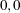#### The Moran Scatter Plot

The Moran scatter plot (Anselin, 1996) is a useful visual tool for exploratory analysis, because it enables you to assess how similar an observed value is to its neighboring observations. Its horizontal axis is based on the values of the observations and is also known as the response axis. The vertical Y axis is based on the weighted average or spatial lag of the corresponding observation on the horizontal X axis. Note: The term spatial lag in the current context is unrelated to the concept of the semivariogram lag presented in the section Distance Classification.

The Moran scatter plot provides a visual representation of spatial associations in the neighborhood around each observation. You specify a neighborhood size with the LAGDISTANCE= option in the COMPUTE statement. The observations are represented by their standardized values; therefore only nonmissing observations are shown in the plot. For each one of those, the VARIOGRAM procedure computes the weighted average, which is the weighted mean value of its neighbors. Then, the centered weighted average is plotted against the standardized observations. As a result, the scatter plot is centered on the coordinates (), and distances in the plot are expressed in deviations from the origin ().

Depending on their position on the plot, the Moran plot data points express the level of spatial association of each observation with its neighboring ones. Conceptually, these characteristics differentiate the Moran plot from the semivariogram. The latter is typically used in geostatistics to depict spatial associations across the whole domain as a continuous function of a distance metric.

You can find the data points on the Moran scatter plot in any of the four quadrants defined by the horizontal line y = 0 and the vertical line x = 0. Points in the upper right (or high-high) and lower left (or low-low) quadrants indicate positive spatial association of values that are higher and lower than the sample mean, respectively. The lower right (or high-low) and upper left (or low-high) quadrants include observations that exhibit negative spatial association; that is, these observed values carry little similarity to their neighboring ones.

When you use binary, row-averaged weights for the creation of the Moran scatter plot and in autocorrelation statistics, Moran’s I coefficient is equivalent to the regression slope of the Moran scatter plot. That is, when you specify

```PLOTS=MORAN(ROWAVG=ON)
```

in the PROC VARIOGRAM statement and

```AUTOCORR(WEIGHTS=BINARY(ROWAVERAGING))
```

in the COMPUTE statement, then the regression line slope of the Moran scatter plot is the Moran’s I coefficient shown in the section Autocorrelation Statistics Types. In this sense, the Moran’s I coefficient has a global character, whereas the Moran scatter plot provides you with a more detailed exploratory view of the autocorrelation behavior of the individual observations.

This detailed view can reveal outliers with respect to the regression line slope of the Moran scatter plot. Outliers, if present, can function as leverage points that affect the value of Moran’s I coefficient. As noted by Anselin (1996), such extremes indicate local instability in spatial association. This instability can be caused either by problems with the autocorrelation weights matrix or by fine-scale characteristics of the spatial structure, which are beneath the current observation structure.